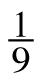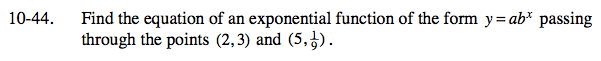### Home > CCA > Chapter 10 > Lesson 10.2.2 > Problem10-44

10-44.

Find the equation of an exponential function of the form y = abx passing through the points (2, 3) and (5,). Homework Help ✎Look at problem 10-33 or the Math Notes box in Lesson 9.3.1 for help solving this problem.

$y=27\left(\frac{1}{3}\right)^\textit{x}$